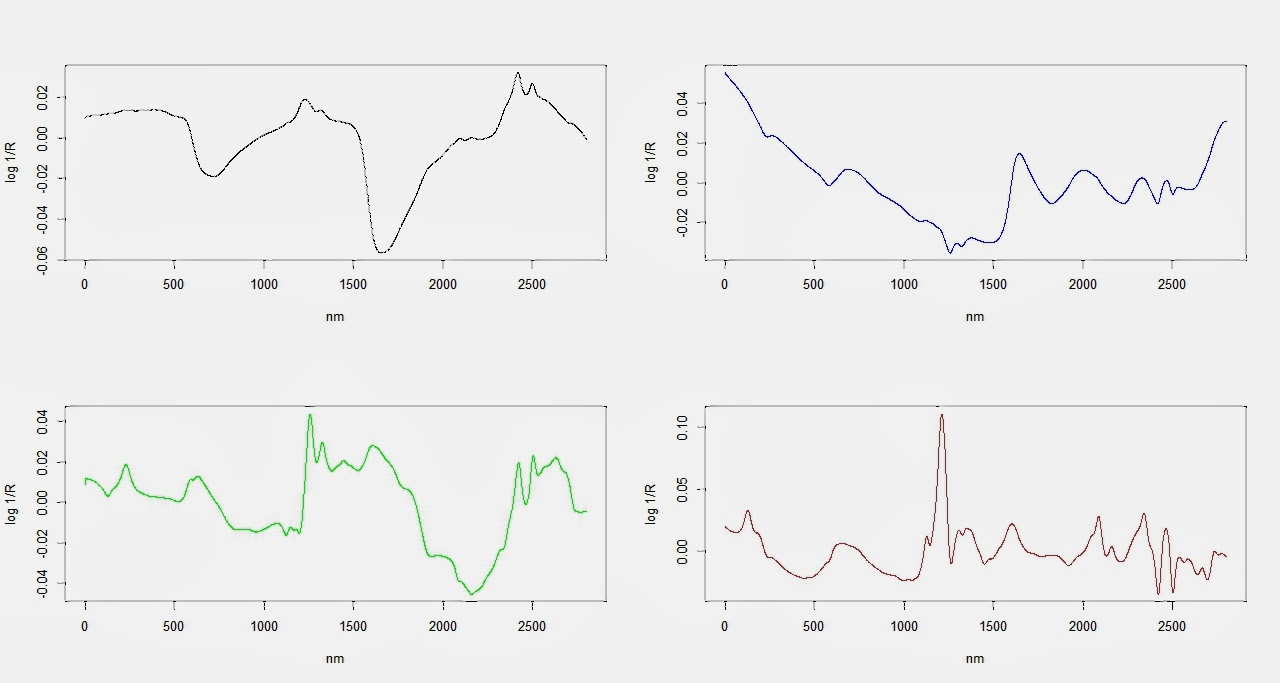## 30 may. 2013

### How do your NIR measurements compare with reference methods?

How do your NIR measurements compare with reference methods?

Try this application available at "nirperformance.com". You can paste yor own  data and get your own results.

## 28 may. 2013

### useR! 2013

Everything seems to be ready for the "R User Conference 2013".
Visit this link for details:
useR! 2013

## 19 may. 2013

### Mahalanobis: "This time with the NIPALS " T " matrix"

Using NIPALS algorithm for PCA, I get two objects:
T : score matrix
P : loadings matrix
library(chemometrics)
sflw.msc3.tra_nipals<-nipals(sflw.msc3.tra\$NIRmsc,a=10,it=160)
"sflw.msc3.tra\$NIRmsc" is my training set spectra treated with MSC.
names(sflw.msc3.tra_nipals)
 "T" "P"
dim(sflw.msc3.tra_nipals\$T)
#T matrix dimension (n . p)
 107  10
n = number of samples
p = number of PCs
dim(sflw.msc3.tra_nipals\$P)
#P matrix dimension (m . p)
 2800   10
m = number of wavelengths
p = number of PCs

Let´s use the drawMahal (classical) function in this case in the T matrix:
T_nipals<-sflw.msc3.tra_nipals\$T
Xn.pc1pc2<-T_nipals[,1:2]
Xn.pc1pc3<-T_nipals[,c(1,3)]
Xn.pc1pc4<-T_nipals[,c(1,4)]
Xn.pc2pc3<-T_nipals[,c(2,3)]
Xn.pc2pc4<-T_nipals[,c(2,4)]
Xn.pc3pc4<-T_nipals[,c(3,4)]
par(mfrow=c(2,3))
drawMahal(Xn.pc1pc2,center=apply(Xn.pc1pc2,2,mean),
covariance=cov(Xn.pc1pc2),quantile=0.975)
drawMahal(Xn.pc1pc3,center=apply(Xn.pc1pc3,2,mean),
covariance=cov(Xn.pc1pc3),quantile=0.975)
drawMahal(Xn.pc1pc4,center=apply(Xn.pc1pc4,2,mean),
covariance=cov(Xn.pc1pc4),quantile=0.975)
drawMahal(Xn.pc2pc3,center=apply(Xn.pc2pc3,2,mean),
covariance=cov(Xn.pc2pc3),quantile=0.975)
drawMahal(Xn.pc2pc4,center=apply(Xn.pc2pc4,2,mean),
covariance=cov(Xn.pc2pc4),quantile=0.975)
drawMahal(Xn.pc3pc4,center=apply(Xn.pc3pc4,2,mean),
covariance=cov(Xn.pc3pc4),quantile=0.975)

To understand better these plots we can have a look to the P (loadings) matrix:
P_nipals<-sflw.msc3.tra_nipals\$P
par(mfrow=c(2,2))
matplot(data.points,P_nipals[,1],type="l",lty=1,xlab="nm",
ylab="log 1/R")
matplot(data.points,P_nipals[,2],type="l",lty=1,xlab="nm",
ylab="log 1/R",col="blue")
matplot(data.points,P_nipals[,3],type="l",lty=1,xlab="nm",
ylab="log 1/R",col="green")
matplot(data.points,P_nipals[,4],type="l",lty=1,xlab="nm",
ylab="log 1/R",col="brown")Looking to the loading plots we can have an idea of which type of variability the loading is representing. In the first and the second (specially in the first one we can see some bands related to the water and fat.## 17 may. 2013

### Code School - Try R

This is a nice page to try R.
As soon as you complete some levels, you unlock others.

Code School - Try R

Enjoy

## 15 may. 2013

### Robust Mahalanobis Ellipse

In this plot we compare the Mahalanobis ellipse, using as center, the mean of the columns and as covariance, the classical covariance (is the same plot than in the previous post, but in this case I added colors and symbols to the samples, according to their sample set), and the Mahalanobis ellipse, based on the robust statistics (in this case the Minimum Covariance Determinant).
The calculation of the MCD is done with the function: covMcd( ). This function gives new robust values: center and cov to use indeed the classical ones.

We see how the distribution of the samples, in the ellipse, change, and also the number of outliers detected.

## 12 may. 2013

### Detecting Outliers (Mahalanobis)

This post is to continue with other post (Median Absolute Deviation). We have our score and loading matrix, and I want to check for outliers. We are going to use the Mahalanobis distance for this purpose, using our score matrix.
What is the dimension of our score matrix in this case. We used 4 PCs, so:
> dim(sflw.msc.rpc\$scores)
 211   4

We have in the rows the samples, and in the columns the scores of the samples for each PC.
We have seen how to plot the pairs for all the combinations of these four PCs, and now, what I want is to draw ellipses based in the Mahalanobis distance to detect outliers.
It is really helpful to have the book “Introduction to Multivariate Statistical Analysis in Chemometrics”,  from Kurt Varmuza and Peter Filzmozer, I recommend really to have it. They have developed the R package “chemometrics”, let´s use it:

>library(chemometrics)

This is a subset for the firsts PCs: PC1 and PC2

>X.pc1pc2<-sflw.msc.rpc\$scores[,1:2]

Now let’s use the function:

>drawMahal(X.pc1pc2,center=apply(X.pc1pc2,2,mean),
+covariance=cov(X.pc1pc2),quantile=0.975)

This plot appears, showing this nice ellipse and some samples out (outliers).

## 10 may. 2013

### ISI Scan: Linking a product to "two different types of PMs"

This video is the reply to one of the followers of this blog. Hope you like:

See also:
Configuring ISI Scan to work with LOCAL

## 8 may. 2013

### Median absolute deviation - Wikipedia, the free encyclopedia

This is a robust statistic to use indeed the standard deviation. You can see in this Wikipedia link details of the theory.
This post is just to see how we can use this statistic with R for the calculation of the Principal Components.
Using the R Library: pcaPP, we use the function:
PCAgrid (x, k = 2, method = c ("mad", "sd", "qn"),.............)
In this function, we select the method to use, beeing "mad", the default one. "X" is our spectral matrix, and "k" is the number of components to compute.
Doing this calculations, we get a matrix with the loadings, and another matrix with the scores, apart from other staistics and values.
To follow some rules let´s call P to our loading matrix, and T to our scores matrix.
library(pcaPP)
sflw.msc.rpc<-PCAgrid(sflw.msc\$NIRmsc,k=4,scale=mad)
P<-sflw.msc.rpc\$loadings
T<-sflw.msc.rpc\$scores
pairs(T[,1:4],col=c("red","blue","green","brown")[sflw.msc\$Set])
I can see the samples used in the calibration set in green color and other comming from diferent instruments in other colors, in order to study the patterns and to know better my database.

Continue this post with: Detecting outliers (Mahalanobis)